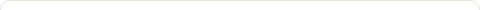# Program to find Sum of Digits in Java

Posted on: February 23, 2016

Objectives :

• Write a program to compute sum of digits of a given number.
• Write a program to find sum of digits of a given number.
• Write a program to calculate sum of digits of a given number.
• Write a program to compute sum of digits of a given integer number.
• Write a program to compute sum of digits of a number entered via Command Line.
• Write a program to compute sum of digits of a given number. Take input from Command Line.
• Write a program to compute sum of digits of a given number. Take input using Scanner class.

Following is the Java Program to compute Sum of Digits of a given integer number;

Method 1 : Java Program to find Sum of Digits when number is entered from command line

```
class SumOfDigits
{
public static void main(String args[])
{
int n;
int a=0;
int sum=0;

//taking integer number from command line and parsing the same
n=Integer.parseInt(args);

while(n!=0)
{
a=n%10;
n=n/10;
sum=sum+a;
}
System.out.println("Sum of digits: " + sum);
}
}

```

Steps to run above program via command line :

1. Compilation : C:\JavaPrograms>javac SumOfDigits.java
2. Interpretation : C:\JavaPrograms>java SumOfDigits 12345

Output : Sum of digits: 15

Method 2 : Java Program to find Sum of Digits if input is taken using Scanner class

```
import java.util.Scanner;

public class SumOfDigits {
public static void main(String args[]) {
Scanner in = new Scanner(System.in);
int n;
int a=0;
System.out.print("Enter a positive number: ");
n = in.nextInt();

if (n <= 0)
System.out.println("You have entered a negative number.");
else {
int sum = 0;

while (n != 0) {

a=n%10;
n=n/10;
sum=sum+a;
}
System.out.println("Sum of digits: " + sum);
}
}
}

```

Steps to run above program via command line :

1. Compilation : C:\JavaPrograms>javac SumOfDigits.java
2. Interpretation : C:\JavaPrograms>java SumOfDigits 12345

Output :

Trial 1 : With positive number

Enter a positive number: 12345

Sum of digits: 15

Trial 2 : With negative number

Enter a positive number: -12345

You have entered a negative number.

### 1 Response to "Program to find Sum of Digits in Java"Yes

LikeComments are closed.

### Email Subscription

Enter your email address to subscribe to this blog and receive notifications of new posts by email.

Join 13,237 other followers